# CBSE Class 12 Chemistry Chapter 4 Revision Notes

Chapter 4: Chemical Kinetics Revision Notes

• Chemical kinetics is a branch of chemistry that studies the rates (or speed) of chemical reactions, as well as the factors that influence them and the mechanisms by which they occur.
• The rate of reaction is the change in reactant or product concentration per unit time.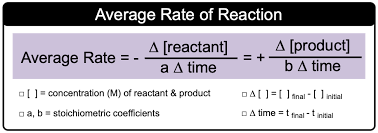Source

• The negative sign denotes a decrease in concentration over time.
• The reaction rate is measured in mol L-1s-1.
• The reaction rate is not a fixed quantity (except for zero order reactions). As the reaction progresses in the forward direction, it decreases.

## Rate Law

A rate law is a mathematical relationship between the reaction rate and one or more reactant’s molar concentration.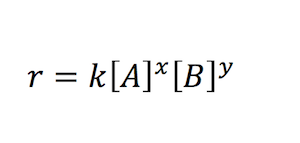Source

• Where x and y are experimentally determined and represent the reaction order with respect to A and B, respectively, x + y represents the overall reaction order.
• The rate constant is the rate of reaction when each reacting species’ concentration is equal. The letter ‘k’ stands for it. It’s also known as the reaction velocity constant or specific reaction rate.

## Factors Affecting Rate of a Chemical Reaction

• Experimental conditions such as concentration of the reactants, nature of the reactants, temperature, etc determine the rate of the reaction.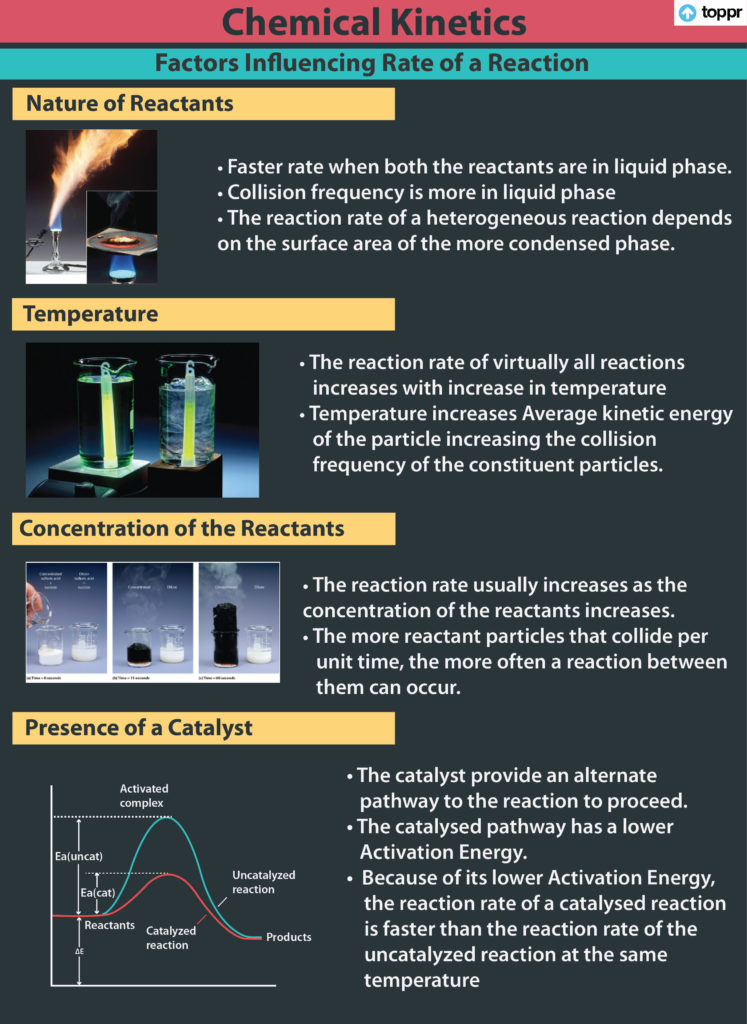Source

## Order and Modularity

• The number of reacting particles (atoms, molecules, or any other species) that collide simultaneously to produce a chemical change is known as modularity of reaction.
• It’s a purely theoretical idea. Its value is a whole number, which is never more than three and it can’t possibly be zero.
• In the rate equation (or rate law) of a reaction, the order of the reaction is defined as the sum of the exponents to which the concentration terms are raised. It can be any whole number, fraction, or zero.
• Zero order reaction: The rate of reactions does not change with concentration of the reactants.
• First order reaction: A first order reaction is one in which the rate of the reaction is determined solely by the variation of one concentration term.
• Second order reaction: the sum of powers of concentration terms is two.
• The integrated rate equation is a function of time that expresses the concentration of reactants.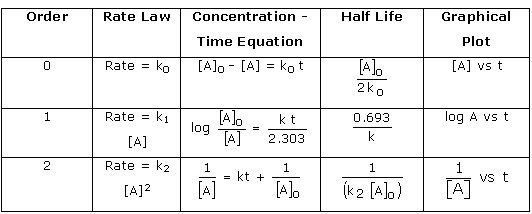Source

• Half-life period: The time it takes for a reactant’s initial concentration to be reduced to half its original value.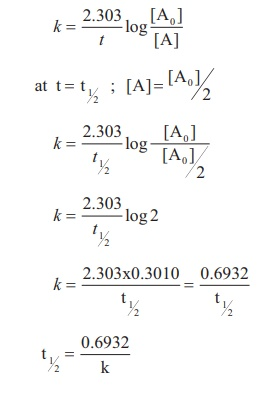Source

• It has been discovered that when the temperature of a chemical reaction rises by 10 degrees Celsius, the rate constant nearly doubles.
• A reaction’s temperature coefficient is the ratio of the rate constants of the reaction at two temperatures that differ by 10°C. The two most common temperatures are 35 °C and 25 °C.
• The Arrhenius equation K=Ae-Ea/Rt can be used to represent the variation of rate constants with temperature, where A is the frequency factor and Ea is the activation energy.
]]>